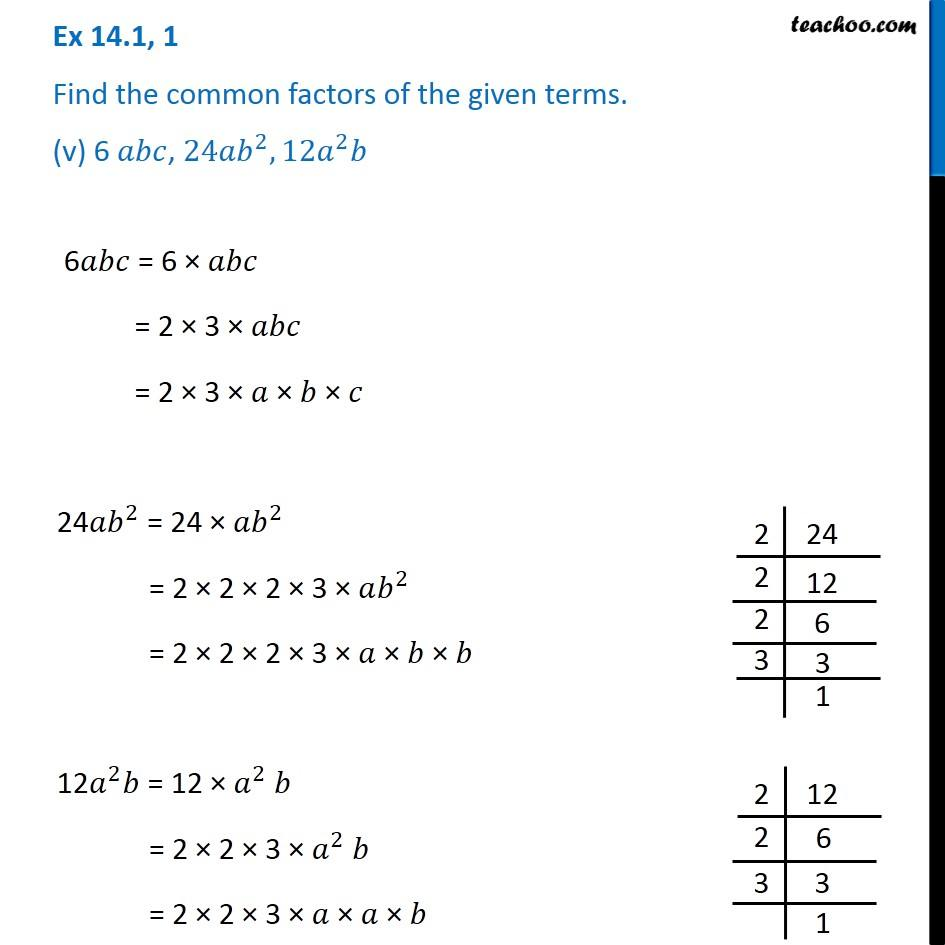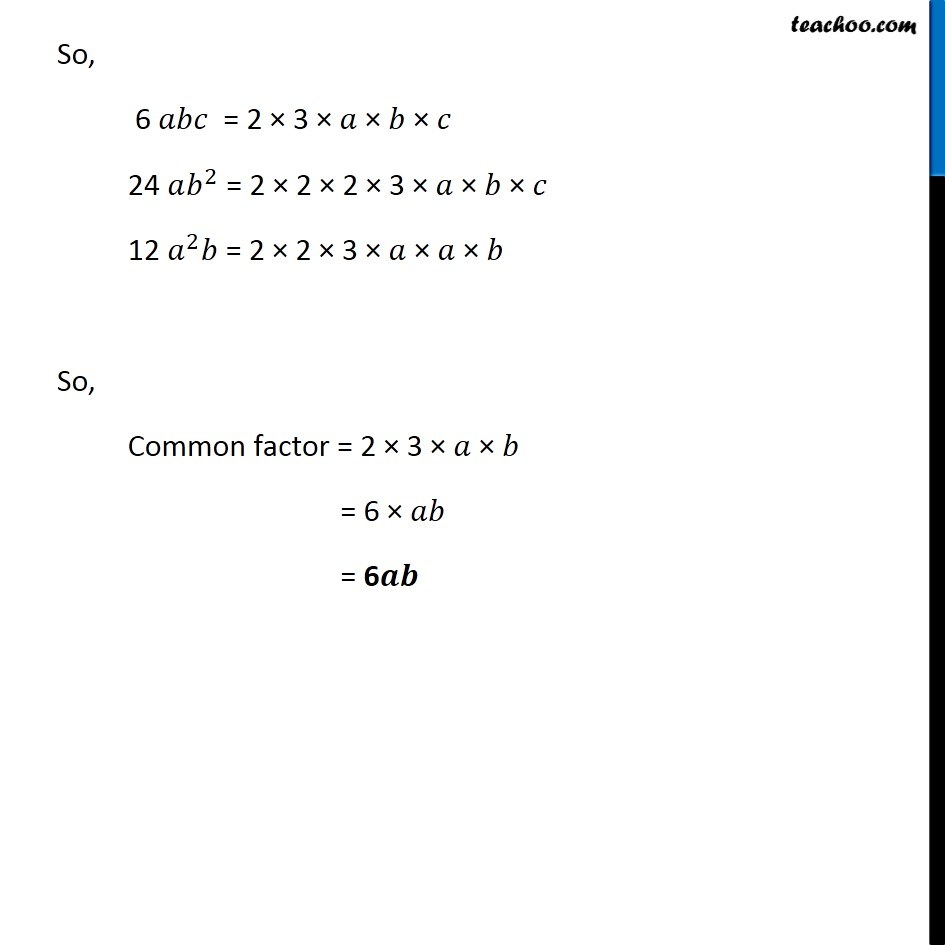Factorisation using common factors

Chapter 12 Class 8 Factorisation
Concept wiseLearn in your speed, with individual attention - Teachoo Maths 1-on-1 Class

### Transcript

Ex 12.1, 1 Find the common factors of the given terms. (v) 6 𝑎𝑏𝑐, 〖24𝑎𝑏〗^2,〖12𝑎〗^2 𝑏 6𝑎𝑏𝑐 = 6 × 𝑎𝑏𝑐 = 2 × 3 × 𝑎𝑏𝑐 = 2 × 3 × 𝑎 × 𝑏 × 𝑐 24〖𝑎𝑏〗^2 = 24 × 〖𝑎𝑏〗^2 = 2 × 2 × 2 × 3 × 𝑎𝑏^2 = 2 × 2 × 2 × 3 × 𝑎 × 𝑏 × 𝑏 12𝑎^2 𝑏 = 12 × 𝑎^2 𝑏 = 2 × 2 × 3 × 𝑎^2 𝑏 = 2 × 2 × 3 × 𝑎 × 𝑎 × 𝑏 So, 6 𝑎𝑏𝑐 = 2 × 3 × 𝑎 "× " 𝑏 × 𝑐 24 𝑎𝑏^2 = 2 × 2 × 2 × 3 × 𝑎 "× " 𝑏 × 𝑐 12 𝑎^2 𝑏 = 2 × 2 × 3 × 𝑎 × 𝑎 × 𝑏 So, Common factor = 2 × 3 × 𝑎 × 𝑏 = 6 × 𝑎𝑏 = 6𝒂𝒃